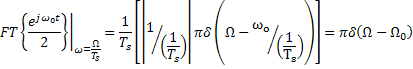# MathWorks Conversations and the FFT

If you are like me, you read the doc, a lot.  I am often clicking on the help just to verify my understanding of a function’s syntax, or the behavior of a block.  That is why I wasn’t surprised to find myself involved in a detailed discussion about the documentation for FFT.

You go it… just another day at the MathWorks.  For some context, the doc example generates a signal corrupted with noise, and then uses the FFT to extract the frequency components.

Fs = 1000;                    % Sampling frequency
T = 1/Fs;                     % Sample time
L = 1000;                     % Length of signal
t = (0:L-1)*T;                % Time vector
% Sum of a 50 Hz sinusoid and a 120 Hz sinusoid
x = 0.7*sin(2*pi*50*t) + sin(2*pi*120*t);
y = x + 2*randn(size(t));     % Sinusoids plus noise
plot(Fs*t(1:50),y(1:50))
title('Signal Corrupted with Zero-Mean Random Noise')
xlabel('time (milliseconds)')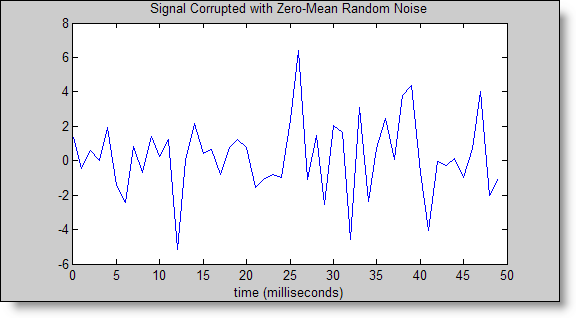NFFT = 2^nextpow2(L); % Next power of 2 from length of y
Y = fft(y,NFFT)/L;
f = Fs/2*linspace(0,1,NFFT/2+1);

% Plot single-sided amplitude spectrum.
plot(f,2*abs(Y(1:NFFT/2+1)))
title('Single-Sided Amplitude Spectrum of y(t)')
xlabel('Frequency (Hz)')
ylabel('|Y(f)|')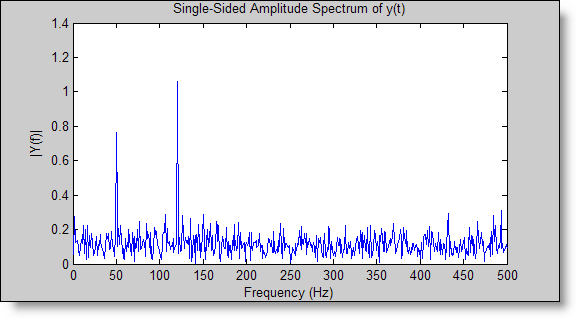There were two questions that started the discussion.  The first one,

Why does the FFT example in the doc have so much code?

We can ignore that for now and focus on the second question, and the real meat of the discussion:

Why does the FFT example result in an amplitude of 1?

My colleague Jeoffrey surprised me with his intimate familiarity with the discrete time Fourier transform and his ability to produce the complicated equations out of thin air.  I told him, “I want that in a blog post!”  So here it is.  Today’s featured guest blogger is Jeoffrey Young:Why does the FFT example result in an amplitude of 1?
By Jeoffrey Young

In this post I will talk about one way of looking at where the scaling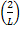comes from in the following example from Matlab 7.7 (R2008b)’s help documentation: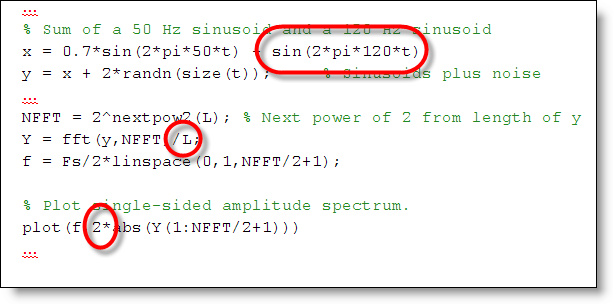To start with, let’s remember that FFT is simply the sampled Discrete Time Fourier Transform of a signal. We know from DTFT that (I’ll use the cosine function here for simplicity):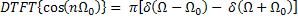where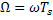and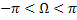(Ever wonder why this looks exactly the same as the Fourier Transform of the continuous time cosine signal? More on this later.) However, it is not enough to sample a continuous time signal that is not time-limited, such as a cosine function. Truncating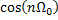so that we can represent the signal on a computer is equivalent to multiplying the time-domain signal by the following rectangular window: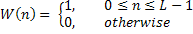The DTFT of this signal is the Digital Sinc Function: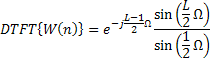Note that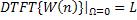by L’Hopital’s Rule.

Thus, from the time-domain multiplication property of Fourier Transform: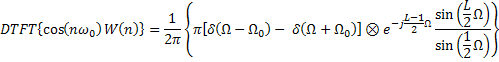Here we see that the DTFT of an L-point truncated cosine function has a maximum amplitude of(if we ignore the effects of aliasing from the overlap and the fact that DTFT is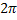periodic).

Question about the scale factor used with FFT are also sent to technical support. This happens often enough that tech support posted a solution titled “Why is the example of using an FFT to get a frequency spectrum scaled the way it is?” We even have a technical note on the general topic of spectral analysis with FFT: Tech Note: 1702 - Using FFT to Obtain Simple Spectral Analysis Plots. As we see it used in this post, the section below describes how the scale factor may also depend on the signal of interest.

Why does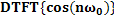looks exactly like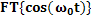?

Remember the following relationship between the DTFT of the sampled analog signal and the FT of the original signal: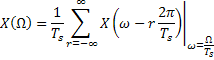In other words, the Discrete Time Fourier transform is scaled by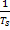. However, the DTFT of a sinusoid is the Delta Function, which is defined more by its properties than its value. Thus, its amplitude has to be scaled when the axis is modified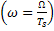: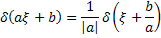So that: